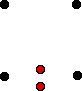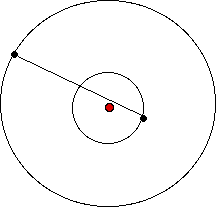Curved Space

There is more there, there.

Introduction

Near a mass, like a star, space becomes "curved." Here is one interesting yet simple thought experiment that shows one property of curved space.

To Do and Notice

Consider four marker buoys in deep space far from any masses. Each buoy sends out light pulses which travel to its neighbors. The neighbors send out a return pulses. The four buoys are adjusted so that the light takes one year to travel from each buoy to its nearest neighbors. The angles are surveyed so that the buoys make a square.Four buoys are arranged at the corners of a square one light year on a side.

Tow two neutron stars into position on either side of the middle of the line between the bottom two buoys.Two neutron stars are placed on either side of the bottom of the square.

Once again the square is surveyed.

The light now takes longer to travel between the bottom two buoys.

The light traveling between the bottom two buoys still appears to travel in a straight line.
The lines between the buoys still make 90 degree angles at the corners.

Yet the bottom distance is longer than the other three distances.

Bringing the stars into position makes more distance between the bottom two stars.

The laws about squares we have learned from Euclidean geometry on earth no longer apply because space is "curved" by the presence of mass.

So What?

A version of this experiment has been done by sending radio signals between antennas on the surface of the earth and the Viking Lander spacecraft on the surface of Mars. The distance to Mars can be precisely measured (to +- 5 m or 1 part in 10^11 ) and its orbit exactly determined. The orbit of the earth is also known precisely. When the radio signals travel near the sun on their way from the earth to Mars and back they take longer because space is curved near the sun, there is more "there" there.A radio signal travels from the earth to mars and back passing close to the sun.

In the case of the Mars spacecraft experiment the light path is also bent into an arc. That is why I used two neutron stars to keep the light path exactly straight. However, the distance along the curved light trajectory is even longer than the extra distance because of the curvature of the path.

Math Root

The distance that light falls under gravity can be easily approximated, just calculate the distance light would fall under the laws of Newtonian mechanics and double the answer. (This approximation only holds when gravity is not supper strong so you cannot use it near black holes and neutron stars.)

So how far, d, does light fall when traveling across a 10 meter wide classroom?

The distance an object fall under Newtonian constant gravitational acceleration, g, in a time, t is:

d = 1/2 g t^2

So we need to know the time it takes light to cross the room, D, since light travels at constant speed c we can calculate the time.

t = D/c = 10 m/ 3 x 10^8 m/s = 3 x 10^-8 s, or 30 nanoseconds.

in 30 nanoseconds light then falls

d = 1/2 * 10 m/s^2 * (3 * 10^-8)^2

d = 5 * 10^-15 m

This is about five times the diameter of a hydrogen nucleus.

The distance that light falls according to general relativity is twice this or 10^-14 m.

Etc.

This was predicted by Einstein. Sir Arthur Eddington photographed stars behind the sun during a total solar eclipse in 1919 the stars appeared to shift position in the sky due to the bending of their light as it passed near the sun. The bending was closer to Einstein's prediction than Newtons. This was one of the first strong experimental confirmations of a prediction of general relativity.